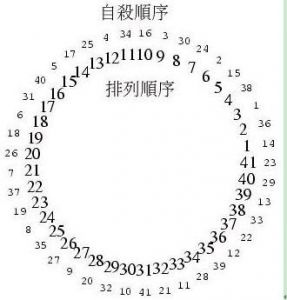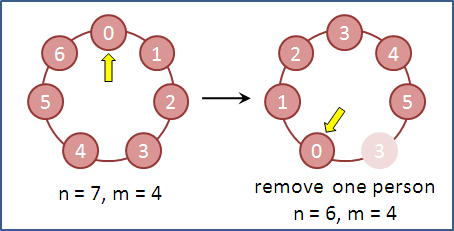## 方法一、直接模拟求解14 36 1 38 15 2 24 30 3 16 34 4 25 17 5 40 31 6 18 26 7 37 19 8 35 27 9 20 32 10 41 21 11 28 39 12 22 33 13 29 23

 `````` 1 2 3 4 5 6 7 8 9 10 11 12 13 14 15 16 17 18 19 20 21 22 23 24 25 26 27 28 29 30 31 32 33 34 35 36 37 38 39 40 41 42 `````` ``````#include #include #define N 41 #define M 3 int main(void) { int man[N] = {0}; int count = 1; int i = 0, pos = -1; int alive = 0; while(count <= N) { do { pos = (pos+1) % N; // 环状处理 if(man[pos] == 0) i++; if(i == M) { // 报数为3了 i = 0; break; } } while(1); man[pos] = count; count++; } printf("\n约琴夫排列："); for(i = 0; i < N; i++) printf("%d ", man[i]); printf("\n\n您想要救多少人？"); scanf("%d", &alive); printf("\nL表示这%d人要放的位置：\n", alive); for(i = 0; i < N; i++) { if(man[i] <= N - alive) printf("D"); else printf("L"); if((i+1) % 5 == 0) printf(" "); } printf("\n"); return 0; } ``````

## 方法二、动态规划

http://en.wikipedia.org/wiki/Josephus_problem``````f(n, m) = (f(n - 1, m) + m) % n
f(1, m) = 0
``````
 ``````1 2 3 4 5 6 7 `````` ``````void josephus(int n, int m) { int answer = 0; for (int i=1; i<=n; ++i) answer = (answer + m) % i; cout << "存活的人是" << answer << "号" << endl; } ``````